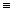# Giorgi Lekishvili and Johann Gasteiger

Computer-Chemie-Centrum, University of Erlangen-Nuremberg, D-91052 Erlangen, Germany

In the past few years some descriptors were designed that contained not only considering the molecular structure, but also had free parameters, or variables. The numerical values of such free parameters are by no means dependent on the molecular structure but have to be optimised to achieve maximal performance of the model. The success of employing this approach crucially depends on the two following points: a refined strategy of how to vary the free parameters, as this must not be done by hand, and a substantial mathematical proof that the models obtained are not simply the by-chance ones fit for the particular dataset.

This work presents a generalized form of the self-optimising indices. Our approach is based on a modern part of mathematics, the lambda calculus.

Let B be the basis of a structural representation of a molecule, such as the adjacency matrix/connectivity table, or a vector containing the number of occurrences of different substructures in the molecule, etc. Let T be an expression possibly containing B. Then, a self-optimising index is a lambda function F of B: lB.T(B).  Here l is the so-called abstractor. For the particular case, i.e., given a numerical value of B for the molecule M, bM, the expression is reduced to a numerical value, DescrM, as shown below:

(lB.T(B))bM->F(M)DescrM

In a simplified case, one has to have a basic form for the T expression, which will be further optimised for particular tasks. In our studies, we have applied the autocorrelation polynomial as the expression and simulated annealing as a technique to optimise it. Alternatively, neural networks could be applied. Thereafter, one can decrease the number of candidate descriptors from several hundreds to less than ten polynomials.

In the most profound case, the genetic programming can be applied to find the optimal expression of the self-optimising descriptor.